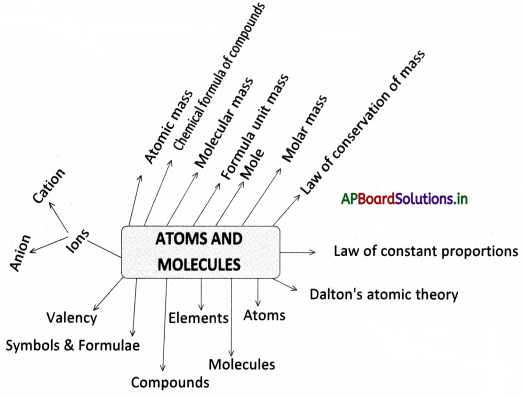# AP Board 9th Class Physical Science Notes Chapter 4 Atoms and Molecules

Students can go through AP State Board 9th Class Physical Science Notes Chapter 4 Atoms and Molecules to understand and remember the concept easily.

## AP State Board Syllabus 9th Class Physical Science Notes Chapter 4 Atoms and Molecules

→ Mass is neither created nor destroyed during a chemical reaction. This is the law of conservation of mass, established by Antoine Lavoisier.

→ A given chemical substance always contains the same elements combined in a fixed proportion by weight. This is the law of constant proportions, proposed by Joseph L. Proust.

→ Dalton proposed atomic theory. According to this, matter consists of indivisible particles called atoms.

→ Atoms combine to form molecules.

→ Atoms of the same elements combine to form molecules.

→ Atoms of different elements combine to form compounds.→ Symbols represent elements and formula represents compounds or molecules.

→ A symbol can have either one or two letters of English.

→ The number of atoms constituting a molecule is known as its atomicity.

→ The combining capacity of an element with another element is known as its valency.

→ Ions are single charged atoms or a group of atoms that have a net charge on them.

→ A positively charged ion is a cation and a negatively charged ion is an anion.

→ Scientists use the relative atomic mass scale to compare the masses of different atoms of elements.

→ The number of times one atom of a given element is heavier than 1/12th part of the atomic mass of carbon -12 is called atomic mass.

→ By using the criss-cross method, we can write the chemical formula of compounds.

→ The molecular mass of a substance is the sum of the atomic masses of all the atoms in a molecule of a substance.→ The formula unit mass of a substance is a sum of atomic masses of all atoms in a formula unit of a compound.

→ One mole of any species is the quantity that is expressed in a number having a mass equal to its atomic or molecular mass in grams.

→ The number of particles present in one mole of any substance is the Avogadro number (Na) which is equal to 6.022 × 1023.

→ The mass of 1 mole of a substance which is expressed in grams is called its molar mass.

→ Law of conservation of mass: The mass of reactants is equal to the mass of the products of chemical reactions.
(or)
Mass is neither created nor destroyed during a chemical reaction.

→ Law of constant proportion: A given chemical substance always contains the same elements combined in a fixed proportion by weight.

→ Atom: An atom is the smallest particle of an element that can participate in a chemical reaction and retain all its properties.

→ Symbol: Symbol is the short form of representing an element.

→ Atomic mass: The relative atomic mass of the atom of an element is defined as the average mass of the atom, as compared to 1/12th of the mass of one carbon – 12 atom.

→ Atomic mass unit (AMU): One atomic mass unit is defined as mass exactly one-twelfth the atomic mass of carbon – 12 isotopes.→ Unified mass (u): Unified mass is the unit of atomic mass used in the place of AMU.

→ Molecule: A molecule can be defined as the smallest particle of matter that is capable of independent existence and retained all the properties of that substance.

→ Molecules of elements: Atoms of the same elements or of different elements can join together to form molecules.

→ Molecules of compounds: Atoms of different elements join together they form new substances known as compounds.

→ Formula: Formula is the shortest form to represent a compound.

→ Ion (Cation and Anion): Ion may be a single charged atom or a group of atoms that have a net charge on them. A positively charged ion is called a cation and a negatively charged ion is called an anion.

→ Valency: Every element reacts with other elements according to its combining capacity, which we call its valency.

→ Molecular mass: The molecular mass of substances is the sum of the atomic masses of all atoms in a molecule of a substance.

→ Formula unit mass: The formula unit mass of a substance Is a sum of the atomic masses of all atoms in a formula unit of a compound.

→ Mole: One mole of any species is the quantity that is expressed in a number having a mass equal to its atomic or molecular mass in grams.→ Avogadro constant: The number of particles present in one mole of any substance is the Avogadro constant (NA). This is equal to 6.022 × 1023.

→ Molar mass: The mass of 1 mole of a substance which is expressed in grams is called its molar mass.→ Antoine Lavoisier:

• HewasaFrench nobleman. He made many important contributions to chemistry and some call him the Father of Modern Chemistry.
• Lavoisier studied combustion reactions in detail. For example, during combustion reactions, he not only carefully weighed the solid reactants but also took into account the gases involved. He perfected chemical apparatus which ensured that gases did not escape during the reactions. This led to the law of conservation of mass.